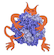IMP  2.4.0 The Integrative Modeling Platform
score_functor Directory ReferenceDirectory dependency graph for score_functor:

## Files

A Score on the distance between a pair of particles.

file  distance_pair_score_macros.h [code]
Various important macros for implementing decorators.

file  score_functor/DistancePairScore.h [code]
A Score on the distance between a pair of particles.

file  Dope.h [code]
A Score on the distance between a pair of particles.

file  score_functor/Harmonic.h [code]
A Score on the distance between a pair of particles.

file  score_functor/HarmonicLowerBound.h [code]
A Score on the distance between a pair of particles.

file  score_functor/HarmonicUpperBound.h [code]
A Score on the distance between a pair of particles.

file  LinearLowerBound.h [code]
A Score on the distance between a pair of particles.

file  score_functor/OpenCubicSpline.h [code]
A Score on the distance between a pair of particles.

file  OrientedSoap.h [code]
Score a particle pair using an orientation-dependent SOAP potential.

file  Score.h [code]
A Score on the distance between a pair of particles.

file  score_functor_config.h [code]

file  ScoreUnaryFunction.h [code]
A Score on the distance between a pair of particles.

file  Shift.h [code]
A Score on the distance between a pair of particles.

file  SingletonStatistical.h [code]
statistical score for a single particle based on one parameter, such as solvent accessibility

file  Soap.h [code]
A Score on the distance between a pair of particles.

file  SphereDistance.h [code]
A Score on the distance between a pair of particles.

file  Statistical.h [code]
A Score on the distance between a pair of particles.

file  UnaryFunctionEvaluate.h [code]
A Score on the distance between a pair of particles.

file  WeightScore.h [code]
A Score on the distance between a pair of particles.